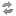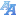Exam dates(version: 207)
Browse before 2023/2024 winter
Login via CASType: all courses ---Institute of the History of Charles University and Archive of Charles UniversityCentre for Theoretical Study;Environment CentreCentre for Knowledge and Technology TransferCatholic Theological FacultyProtestant Theological FacultyHussite Theological FacultyFaculty of LawFirst Faculty of MedicineSecond Faculty of MedicineThird Faculty of MedicineFaculty of Medicine in PilsenFaculty of Medicine in Hradec KrálovéFaculty of Pharmacy in Hradec KrálovéFaculty of ArtsFaculty of ScienceFaculty of Mathematics and PhysicsFaculty of EducationFaculty of Social SciencesFaculty of Physical Education and SportFaculty of HumanitiesInstitute for Language and Preparatory StudiesCentral Library of Charles UniversityCentre for Economic Research and Graduate EducationRectorate---Astronomical Institute of Charles University (32-AUUK)Blíže nespecifikované praxe (32-#PRX)Computer Science Institute of Charles University (32-IUUK)Department of Algebra (32-KA)Department of Applied Mathematics (32-KAM)Department of Atmospheric Physics (32-KFA)Department of Condensed Matter Physics (32-KFKL)Department of Distributed and Dependable Systems (32-KDSS)Department of Geophysics (32-KG)Department of Chemical Physics and Optics (32-KCHFO)Department of Language Education (32-KJP)Department of Low Temperature Physics (32-KFNT)Department of Macromolecular Physics (32-KMF)Department of Mathematical Analysis (32-KMA)Department of Mathematics Education (32-KDM)Department of Numerical Mathematics (32-KNM)Department of Physical Education (32-KTV)Department of Physics Education (32-KDF)Department of Physics of Materials (32-KFM)Department of Probability and Mathematical Statistics (32-KPMS)Department of Software and Computer Science Education (32-KSVI)Department of Software Engineering (32-KSI)Department of Surface and Plasma Science (32-KFPP)Department of Theoretical Computer Science and Mathematical Logic (32-KTIML)Institute of Formal and Applied Linguistics (32-UFAL)Institute of Mathematics CAS (32-MUAV)Institute of Particle and Nuclear Physics (32-UCJF)Institute of Physics of Charles University (32-FUUK)Institute of Theoretical Physics (32-UTF)Laboratory of General Physics Education (32-KVOF)Mathematical Institute of Charles University (32-MUUK)Network and Labs Management Center (32-SISAL)Student Affairs Department (32-STUD)---Selected Topics on Mathematics for Physicists - NMAF006Advanced Linear Algebra for Physicists - NMAF037Open Problem Seminar - NMAT057Seminar for bachelor thesis - NMAT362Mathematical Analysis 1 - NMMA101Mathematical Analysis 2 - NMMA102Calculus 2 - NMMA122Proseminar on Mathematical Analysis - NMMA161Proseminar on Mathematical Analysis - NMMA162Metric Spaces - NMMA164Mathematical Analysis 3 - NMMA201Mathematical Analysis 4 - NMMA204Measure and Integration Theory - NMMA205Calculus 2 - NMMA221Proseminar on Mathematical Analysis 3 - NMMA261Proseminar on Mathematical Analysis 4 - NMMA263Introduction to Complex Analysis - NMMA301Introduction to Functional Analysis - NMMA331Ordinary Differential Equations - NMMA336Seminar on Real Functions Theory 1 - NMMA337Introduction to Partial Differential Equations - NMMA339Seminar on Real Functions Theory 2 - NMMA340Calculus 3 - NMMA341Selected Topics on Functional Analysis - NMMA342Measure and Integral Theory 2 - NMMA343General Topology 1 - NMMA345Seminar on Basic Properties of Function Spaces - NMMA347Seminar on Basic Properties of Function Spaces - NMMA348Metric Structures - NMMA361Topology of a continuum - NMMA363Functional Analysis 1 - NMMA401Functional Analysis 2 - NMMA402Theory of Real Functions 1 - NMMA403Ordinary Differential Equations 2 - NMMA407Complex Analysis - NMMA410Seminar on Differential Equations - NMMA431Descriptive Set Theory 1 - NMMA433Descriptive Set Theory 2 - NMMA434Advanced Differentiation and Integration 1 - NMMA437Advanced Differentiation and Integration 2 - NMMA438Differential Equations in Banach Spaces - NMMA440Seminar on Function Spaces - NMMA454Seminar on Real and Abstract Analysis - NMMA455Seminar on Real Functions Theory - NMMA456Seminar on Basic Properties of Function Spaces - NMMA457Seminar on Topology - NMMA458Seminar on Fundamentals of Functional Analysis - NMMA459General Topology 2 - NMMA462Problem solving seminar - NMMA465Applications of Differential Equations in Biology - NMMA466An introduction to mathematical homogenization - NMMA469Chapters from discrete dynamical systems - NMMA479Selected topics in harmonic analysis 1 - NMMA481Selected topics in harmonic analysis 2 - NMMA482Nonlinear Functional Analysis 1 - NMMA501Nonlinear Functional Analysis 2 - NMMA502Operator Algebras 1 - NMMA561Operator Algebras 2 - NMMA562Introduction to Approximation Theory 1 - NMMA565Introduction to Approximation Theory 2 - NMMA566Introduction to asymptotic analysis for integrable equations 1 - NMMA569Topics in Dynamical Systems - NMMA574Mappings of finite distortion 1 - NMMA577Mappings of finite distortion 2 - NMMA578Regularity of Weak Solutions to Partial Differential Equations - NMMA584Introduction to asymptotic analysis for integrable equations 2 - NMMA586Applied differential equations - NMMA706Introduction to Complex Analysis (O) - NMMA901Introduction to Functional Analysis (O) - NMMA931Selected Topics on Functional Analysis (O) - NMMA942--- only future only the free ones 20501002505001000
* no data found

Charles University | Information system of Charles University | http://www.cuni.cz/UKEN-329.html